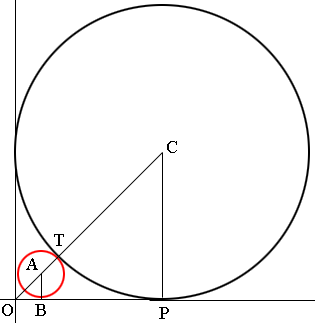SEARCH HOMEMath Central Quandaries & QueriesDaryl, a student: Find the equation of the smaller circle that is tangent to the axes and the circle x2+y2=2x+2y-1?Hi Daryl,

If you put the circle equation in standard form you will see that is the circle with centre (1, 1) and radius 1. That's the black circle in my diagram.Hence |OP| = |PC| = 1 and using Pythagoras theorem |CO| = sqrt(2).
Let r be the radius of the small circle.
Triangle COP and AOB are similar and hence 1/sqrt(2) = |BA|/|AO|
But |BA| = r and |AO| = |CO| - (|CT| + |TA|). Substitute into the equation on the previous line and solve for r.

PennyMath Central is supported by the University of Regina and The Pacific Institute for the Mathematical Sciences.